# 2.   Use number line and add the following integers :             (a)               (b)              (c)              (d)              (e)               (f)

M manish painkra

Addition by using the number line method-
(a)
Initially, move 0 to 9 and then 6 number left to the 9 on the nu,ber line.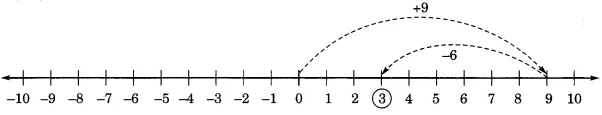(b)
Initially, move 0 to 5 and then 11 number left to the 5 on the number line.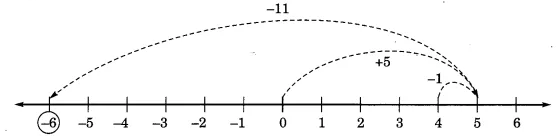(c)
Initially, move 0 to -1 and then 7 number left to the -1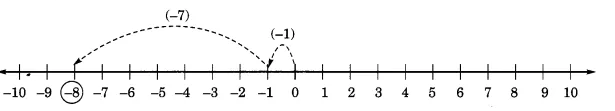(d)
Initially, move 0 to -5 and then 2 number right to the -5 and again moves 10 number right to the -5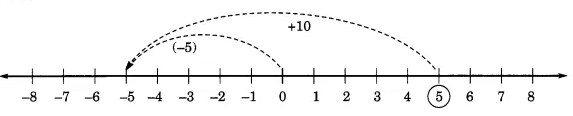(e)
Initially, move 0 to -1 and then 2 number left to the -1 and again moves 3 number left to the -3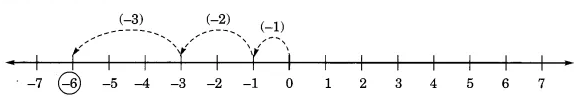(f)
First move 0 to -2 and then -2 to 8 number right on the number line and then move 4 numbers left to the 6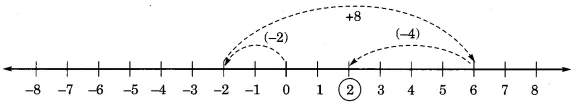Exams
Articles
Questions